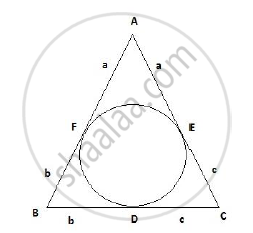# The incircle of an isosceles triangle ABC, in which AB = AC, touches the sides BC, CA and AB at D, E and F respectively. Prove that BD = DC. - Mathematics

The incircle of an isosceles triangle ABC, in which AB = AC, touches the sides BC, CA and AB at D, E and F respectively. Prove that BD = DC.

#### SolutionGiven: ABC is an isosceles triangle in which AB = AC; with a circle inscribed
in the triangle.
To prove: BD = DC
Proof:
AF and AE are tangents drawn to the circle from point A.
Since two tangents drawn to a circle from the same exterior point are equal,
AF = AE = a
Similarly BF = BD = b and CD = CE = c
We also know that Δ ABC is an isosceles triangle in which AB = AC.
a+b = a+c
Thus b = c
Therefore, BD = DC
Hence proved.

Concept: Triangles Examples and Solutions
Is there an error in this question or solution?
2013-2014 (March) All India Set 2

Share